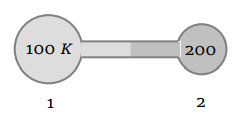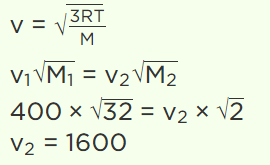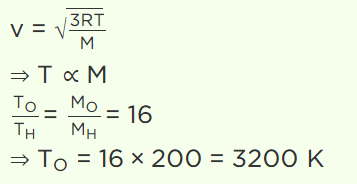## Kinetic Theory Questions and Answers Part-10

1. Equation of gas in terms of pressure (P), absolute temperature (T) and density (d) is
a) $\frac{P_{1}}{T_{1}d_{1}}=\frac{P_{2}}{T_{2}d_{2}}$
b) $\frac{P_{1}T_{1}}{d_{1}}=\frac{P_{2}T_{2}}{d_{2}}$
c) $\frac{P_{1}d_{2}}{T_{1}}=\frac{P_{2}d_{1}}{T_{1}}$
d) $\frac{P_{1}d_{1}}{T_{1}}=\frac{P_{2}d_{2}}{T_{2}}$

Explanation:2. At constant pressure, the ratio of increase in volume of an ideal gas per degree raise in kelvin temperature to it's original volume is (T = absolute temperature of the gas)
a) T
b) $T^{2}$
c) $\frac{1}{T}$
d) $\frac{1}{T^{2}}$

Explanation: At constant pressure, the ratio of increase in volume of an ideal gas per degree raise in kelvin temperature to it's original volume is $\frac{1}{T}$

3. Figure shows two flasks connected to each other. The volume of the flask 1 is twice that of flask 2. The system is filled with an ideal gas at temperature 100 K and 200 K respectively. If the mass of the gas in 1 be m then what is the mass of the gas in flask 2a) m
b) $\frac{m}{2}$
c) $\frac{m}{4}$
d) $\frac{m}{8}$

Explanation: $\frac{m}{4}$

4. If the molecular weight of two gases are $m_{1}$  and $m_{2}$  , then at a temperature the ratio of root mean square velocity $v_{1}$  and $v_{2}$  will be
a) $\sqrt{\frac{m_{1}}{m_{2}}}$
b) $\sqrt{\frac{m_{2}}{m_{1}}}$
c) $\sqrt{\frac{m_{1}+m_{2}}{m_{1}-m_{2}}}$
d) $\sqrt{\frac{m_{1}-m_{2}}{m_{1}+m_{2}}}$

Explanation:5. To what temperature should the hydrogen at 327°C be cooled at constant pressure, so that the root mean square velocity of its molecules become half of its previous value
a) – 123°C
b) 123°C
c) – 100°C
d) 0°C

Explanation:6. At a certain temperature, the r.m.s. velocity for $O_{2}$ is 400 m/sec. At the same temperature, the r.m.s. velocity for $H_{2}$ molecules will be
a) 100 m/sec
b) 25 m/sec
c) 1600 m/sec
d) 6400 m/sec

Explanation:7. At a pressure of $24 \times10^{5}dyne\diagup cm^{2}$  , the volume of $O_{2}$ is 10 litre and mass is 20 gm. The r.m.s. velocity will be
a) 800 m/sec
b) 400 m/sec
c) 600 m/sec
d) Data is incomplete

Explanation:8. The r.m.s. velocity will be greater for
a) Hydrogen
b) Oxygen
c) Equal for both
d) Nothing is definite

Explanation: The r.m.s. velocity will be greater for hydrogen

9. In thermal equilibrium, the average velocity of gas molecules is
a) Proportional to $\sqrt{T}$
b) Proportional to $T^{2}$
c) Proportional to $T^{3}$
d) Zero

Explanation: In thermal equilibrium, the average velocity of gas molecules is proportional to$\sqrt{T}$